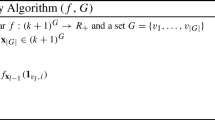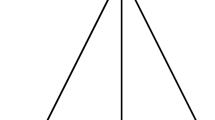# A compact representation for minimizers of k-submodular functions

## Abstract

A k-submodular function is a generalization of submodular and bisubmodular functions. This paper establishes a compact representation for minimizers of a k-submodular function by a poset with inconsistent pairs (PIP). This is a generalization of Ando–Fujishige’s signed poset representation for minimizers of a bisubmodular function. We completely characterize the class of PIPs (elementary PIPs) arising from k-submodular functions. We give algorithms to construct the elementary PIP of minimizers of a k-submodular function f for three cases: (i) a minimizing oracle of f is available, (ii) f is network-representable, and (iii) f arises from a Potts energy function. Furthermore, we provide an efficient enumeration algorithm for all maximal minimizers of a Potts k-submodular function. Our results are applicable to obtain all maximal persistent labelings in actual computer vision problems. We present experimental results for real vision instances.

This is a preview of subscription content, log in via an institution to check access.

Price excludes VAT (USA)
Tax calculation will be finalised during checkout.

### Similar content being viewed by others### A Compact Representation for Minimizers of k-Submodular Functions (Extended Abstract)### On maximizing monotone or non-monotone k-submodular functions with the intersection of knapsack and matroid constraints

Kemin Yu, Min Li, … Qian Liu### Submodular Functions and Rooted Trees

Yaokun Wu & Yinfeng Zhu

## References

• Ando K, Fujishige S (1994) $$\sqcup ,\sqcap$$-closed families and signed posets. Technical report, Forschungsinstitut für Diskrete Mathematik, Universität Bonn

• Ardila F, Owen M, Sullivant S (2012) Geodesics in CAT(0) cubical complexes. Adv Appl Math 48:142–163

• Babenko MA, Karzanov AV (2012) On weighted multicommodity flows in directed networks. arXiv:1212.0224v1

• Barthélemy J-P, Constantin J (1993) Median graphs, parallelism and posets. Discret Math 111(1–3):49–63

• Bouchet A (1997) Multimatroids I. Coverings by independent sets. SIAM J Discret Math 10:626–646

• Chepoi V (2012) Nice labeling problem for event structures: a counterexample. SIAM J Comput 41:715–727

• Feder T (1994) Network flow and 2-satisfiability. Algorithmica 11:291–319

• Fujishige S (1995) Submodular functions and combinatorial optimization (in Japanese). In: Proceedings of the 7th research association of mathematical programming symposium (RAMP 1995), pp 13–28

• Gridchyn I, Kolmogorov V (2013) Potts model, parametric maxflow and $$k$$-submodular functions. In: Proceedings of the IEEE international conference on computer vision (ICCV 2013), pp 2320–2327

• Hirai H (2010) A note on multiflow locking theorem. J Op Res Soc Jpn 53(2):149–156

• Hirai H (2015) L-extendable functions and a proximity scaling algorithm for minimum cost multiflow problem. Discret Optim 18:1–37

• Hirai H, Iwamasa Y (2016) On $$k$$-submodular relaxation. SIAM J Discret Math 30:1726–1736

• Hirai H, Oki T (2016) A compact representation for minimizers of $$k$$-submodular functions (extended abstract). In: Proceedings of the 4th international symposium on combinatorial optimization (ISCO 2016), volume 9849 of lecture notes in computer science, Springer, Cham, pp 381–392

• Huber A, Kolmogorov V (2012) Towards minimizing $$k$$-submodular functions. In: Proceedings of the 2nd international symposium on combinatorial optimization (ISCO 2012), Volume 7422 of lecture notes in computer science, Springer, Heidelberg, pp 451–462

• Ibaraki T, Karzanov AV, Nagamochi H (1998) A fast algorithm for finding a maximum free multiflow in an inner eulerian network and some generalizations. Combinatorica 18:61–83

• Iwamasa Y (2017) On a general framework for network representability in discrete optimization. J Comb Optim. doi:10.1007/s10878-017-0136-y

• Iwata S, Tanigawa S, Yoshida Y (2016a) Improved approximation algorithms for $$k$$-submodular function maximization. In: Proceedings of the 27th annual ACM-SIAM symposium on discrete algorithms (SODA 2016), pp 404–413

• Iwata Y, Wahlström M, Yoshida Y (2016b) Half-integrality, LP-branching and FPT algorithms. SIAM J Comput 45:1377–1411

• Kavvadias DJ, Sideri M, Stavropoulos EC (2000) Generating all maximal models of a Boolean expression. Inf Process Lett 74:157–162

• Kolmogorov V, Thapper J, Živný S (2015) The power of linear programming for general-valued CSPs. SIAM J Comput 44:1–36

• Kovtun I (2003) Partial optimal labeling search for a NP-hard subclass of (max,+) problems. In: Proceedings of the 25th German association for pattern recognition (DAGM 2003), volume 2781 of lecture notes in computer science, Springer, Heidelberg, pp 402–409

• Murota K (2000) Matrices and matroids for systems analysis. Springer, Berlin

• Nielsen M, Plotkin G, Winskel G (1981) Petri nets, event structures and domains, part I. Theor Comput Sci 13:85–108

• Orlin JB (2013) Max flows in $$\text{O}(nm)$$ time, or better. In: Proceedings of the 45th annual ACM Symposium on theory of computing (STOC 2013), pp 765–774

• Picard J-C, Queyranne M (1980) On the structure of all minimum cuts in a network and applications. In: Rayward-Smith VJ (ed) Combinatorial optimization II, volume 13 of mathematical programming studies. Springer, Berlin, pp 8–16. doi:10.1007/BFb0120902

• Reiner V (1993) Signed posets. J Comb Theory Ser A 62:324–360

• Scharstein D, Szeliski R (2003) High-accuracy stereo depth maps using structured light. In: Proceedings of the 2003 IEEE computer society conference on computer vision and pattern recognition (CVPR 2003), pp 195–202

• Scharstein D, Szeliski R, Zabih R (2001) A taxonomy and evaluation of dense two-frame stereo correspondence algorithms. In: Proceedings of the IEEE workshop on stereo and multi-baseline vision (SMBV 2001), pp 131–140

• Sholander M (1954) Medians and betweenness. Proc Am Math Soc 5(5):801–807

• Squire MB (1995) Enumerating the ideals of a poset. Technical report, North Carolina State University

## Acknowledgements

We thank Kazuo Murota, Satoru Fujishige, and the referees for helpful comments. This work was partially supported by JSPS KAKENHI Grant Numbers 25280004, 26330023, 26280004, and by JST, ERATO, Kawarabayashi Large Graph Project.

## Author information

Authors

### Corresponding author

Correspondence to Taihei Oki.